The VARIOGRAM Procedure

Computational Resources

The fundamental computation of the VARIOGRAM procedure is binning: for each pair of observations in the input data set, a distance class and an angle class are determined and recorded. Let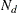denote the number of distance classes,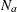denote the number of angle classes, and
denote the number of VAR variables. The memory requirements for these operations are proportional to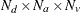. This is typically small.

The CPU time required for the computations is proportional to the number of pairs of observations, or to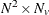, where N is the number of observations in the input data set.